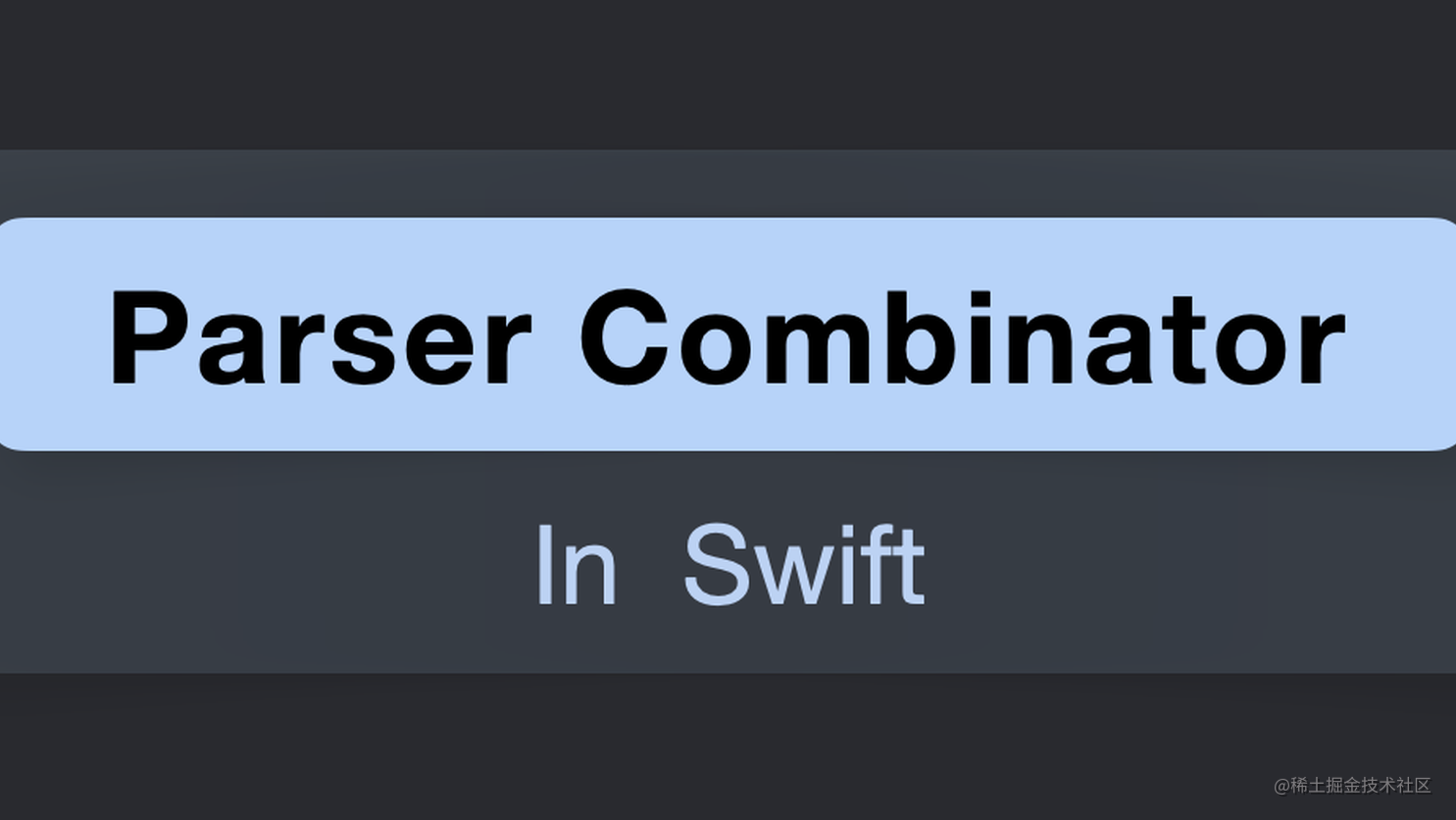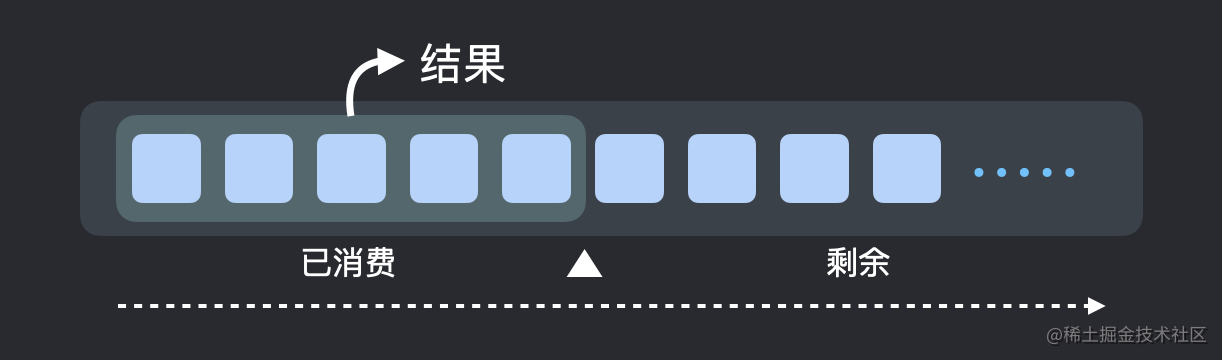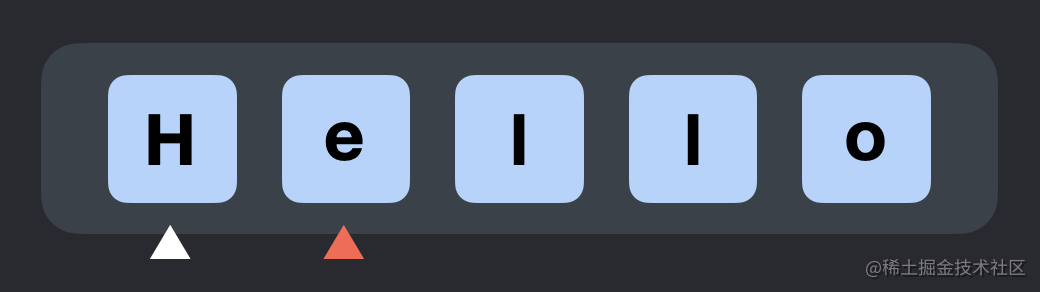函数式编程进阶 - 实现 Parser Combinator [Swift描述]前言

Parser（解析器）能分析输入，产生结果。如正则表达式引擎可以解析匹配输入的字符串、JSONSerialization可帮助 iOS/Mac 开发者将 JSON 解析成 Objective-C 中的 Dictionary。 Parser CombinatorParser的一种实现方式，其基于函数式编程思想，姿态十分优雅。使用它，我们可以非常方便地编写解析逻辑，代码简洁且易于理解。Parser Combinator在设计上充分体现了组合的思想，通过运用其多种Combinator（组合子），我们可以将若干的细粒度子Parser组合在一起以得到更粗粒度的Parser，而后，组合可以继续，抽象度也逐步提升。

模型

基础模型typealias Parser<Value> = (String) -> Result<(Value, String), Error>

Parser在这里被定义成了函数类型，因其解析后输出的结果不定，这里使用了Value泛型来指代结果的类型。函数的输入参数类型为String，返回的是Result。若解析成功，Result则装载了一个二元组，里面的值分别代表了解析的结果以及剩余的输入串；当解析失败时，错误信息也将通过Result装载返回。

typealias Parser = (String) -> Result<(Int, String), Error>

优化模型

typealias Parser<Value> = (Context) -> Result<String, Error>

实现

Context

public final class Context {

public typealias Stream = String
public typealias Element = Stream.Element
public typealias Index = Stream.Index

public let stream: Stream

public init(stream: Stream) {
self.stream = stream
_cursor = stream.startIndex
}

private var _cursor: Index
}

Context还对外提供了消费方法：

// MARK: - Iterator

extension Context: IteratorProtocol {

public func next() -> Element? {
let range = stream.startIndex..<stream.endIndex
guard range.contains(_cursor) else {
return nil
}
defer {
stream.formIndex(after: &_cursor)
}
return stream[_cursor]
}
}

Error

public struct Error: Swift.Error {

public let stream: Context.Stream
public let position: Context.Index
public let message: String

public init(stream: Context.Stream, position: Context.Index, message: String) {
self.stream = stream
self.position = position
self.message = message
}
}

Error记录了输入串、输入串当前所解析到的位置以及以字符串类型表示的失败信息。当解析失败时，我们能通过Parser返回的Result获取到Error的实例，从而了解到失败的原因，以及当前解析所在的位置。

// MARK: - Error

public extension Context {

func `throw`<T>(_ errorMessage: String) -> Result<T, Error> {
.failure(error(with: errorMessage))
}

func error(with message: Stream) -> Error {
.init(stream: stream, position: _cursor, message: message)
}
}

Parser

typealias Parser<Value> = (Context) -> Result<String, Error>

public struct Parser<Value> {

public typealias Result = Swift.Result<Value, Error>

public let parse: (Context) -> Result

public init(_ parse: @escaping (Context) -> Result) {
self.parse = parse
}
}

public extension Parser {

func parse(_ stream: Context.Stream) -> Result {
parse(.init(stream: stream))
}
}

初尝 Parser

OK，目前我们基本上已经定义好了Parser Combinator库核心的几个数据结构，接下来先试玩一下：

public let element = Parser<Context.Element> {
if let element = \$0.next() {
return .success(element)
} else {
return \$0.throw("unexpected end of stream")
}
}

public let endOfStream = Parser<()> {
if \$0.next() == nil {
return .success(())
} else {
return \$0.throw("expecting end of stream")
}
}

• element：在解析时通过Context消费一个输入串元素（字符），如果输入串在之前已经被消费完了，则返回错误。
• endOfStream：跟element一样会通过Context消费输入串，但是它预期的是输入串在之前已经被消费完毕，否则返回错误。

let inputOne = "hello world!"
let inputTwo = ""

let inputOneResultOfElement = element.parse(inputOne)
let inputTwoResultOfElement = element.parse(inputTwo)

let inputOneResultOfEndOfStream = endOfStream.parse(inputOne)
let inputTwoResultOfEndOfStream = endOfStream.parse(inputTwo)

inputOneResultOfElement: success("h")

inputTwoResultOfElement: failure(Error(stream: "", position: Swift.String.Index(_rawBits: 1), message: "unexpected end of stream"))

inputOneResultOfEndOfStream: failure(Error(stream: "hello world!", position: Swift.String.Index(_rawBits: 65793), message: "expecting end of stream"))

inputTwoResultOfEndOfStream: success()

Combinator

public extension Parser {

static func just(_ value: Value) -> Parser<Value> {
.init { _ in .success(value) }
}

func flatMap<O>(_ transform: @escaping (Value) -> Parser<O>) -> Parser<O> {
.init {
switch self.parse(\$0) {
case .success(let value):
return transform(value).parse(\$0)
case .failure(let error):
return .failure(error)
}
}
}
}

• just方法返回的Parser，其解析逻辑不消费任何输入串，只会将just的输入参数直接包装到Result中，作为解析结果返回。

• flatMap里，我们会先运行当前Parser的解析逻辑，若解析成功，解析的结果将作为参数传入flatMap的闭包参数transform中；闭包调用将返回一个新的Parser，而后这个新Parser的解析逻辑将会继续运行；若当前Parser解析失败，错误信息将直接从flatMap返回。

justflatMap主要是针对解析成功后的结果进行映射，对于解析失败的情况，我们也能写出相对应的Combinator:

public extension Parser {

static func error<T>(_ error: Error) -> Parser<T> {
.init { _ in .failure(error) }
}

static func `throw`(_ errorMessage: String) -> Parser<Value> {
.init { \$0.throw(errorMessage) }
}

func flatMapError(_ transform: @escaping (Error) -> Parser<Value>) -> Parser<Value> {
.init {
switch self.parse(\$0) {
case .success(let value):
return .success(value)
case .failure(let error):
return transform(error).parse(\$0)
}
}
}
}

Functor

public extension Parser {

func map<O>(_ transform: @escaping (Value) -> O) -> Parser<O> {
flatMap {
.just(transform(\$0))
}
}
}

map方法做的事情是对当前Parser解析后的结果做映射变换，就类似于 Swift 中对数组进行map操作：let arr = [1,2,3,4].map { \$0 + 1 }

flatMap类似，map亦可编写针对错误情况的版本：

public extension Parser {

func mapError(_ transform: @escaping (Error) -> Error) -> Parser<Value> {
flatMapError {
.error(transform(\$0))
}
}
}

let twoElements = element.flatMap { first in
element.map { second in
String([first, second])
}
}
let result = twoElements.parse("hello")

过滤

Swift 数组具有filter方法，可根据谓词过滤掉不想要的元素。Parser也可以做到类似的效果，我们可以直接为Parser定义一个同名的filter方法：

public extension Parser {

func filter(_ label: String, predicate: @escaping (Value) -> Bool) -> Parser<Value> {
flatMap {
predicate(\$0) ? .just(\$0) : .throw(label)
}
}
}

filter接受两个参数，第一个以字符串为类型，表示谓词判断失败时，返回的错误中所携带的提示信息；第二个则为谓词闭包，通过返回Bool以表示结果是否符合预期。

public extension Parser {

func equal(to value: Value) -> Parser<Value> where Value: Equatable {
filter("expecting \(value)") { \$0 == value }
}

func notEqual(to value: Value) -> Parser<Value> where Value: Equatable {
filter("unexpected \(value)") { \$0 != value }
}
}

let str = "hello"
let resultOne = element.equal(to: "h").parse(str)
let resultTwo = element.notEqual(to: "h").parse(str)

resultOne: success("h")

resultTwo: failure(Error(stream: "hello", position: Swift.String.Index(_rawBits: 65793), message: "unexpected h"))

public func char(_ char: Character) -> Parser<Character> {
element.equal(to: char)
}

public func notChar(_ char: Character) -> Parser<Character> {
element.notEqual(to: char)
}

let str = "hello"
let resultOne = char("h").parse(str)
let resultTwo = notChar("h").parse(str)

public func string(_ string: String) -> Parser<String> {
.init {
for element in string {
if case .failure(let error) = char(element).parse(\$0) {
return .failure(error)
}
}
return .success(string)
}
}

string进行尝试：

let parser = string("Hello")
let resultOne = parser.parse("Helao")

resultOne: failure(Error(stream: "Helao", position: Swift.String.Index(_rawBits: 262401), message: "expecting l"))

resultTwo: success("Hello")

要左不要右、要右不要左

public extension Parser {

func usePrevious<O>(_ next: Parser<O>) -> Parser<Value> {
flatMap { previous in next.map { _ in previous } }
}

func useNext<O>(_ next: Parser<O>) -> Parser<O> {
flatMap { _ in next }
}

func between<L, R>(_ left: Parser<L>, _ right: Parser<R>) -> Parser<Value> {
left.useNext(self).usePrevious(right)
}
}

• left.usePrevious(right)：输入串会依次经过leftright的解析逻辑，但最后会忽略right的解析结果，只返回left的结果。
• left.useNext(right)：输入串会依次经过leftright的解析逻辑，但最后会忽略left的解析结果，只返回right的结果。
• aParser.bwtween(left, right)则会依次经过leftaParserright的解析逻辑，但最后会忽略leftright的解析结果，只返回aParser的结果。

public let charLiteral = element.between(char("'"), char("'"))

let str = "'A'"
let result = charLiteral.parse(str)

选择

public extension Error {

func merge(with another: Error) -> Error {
position > another.position ? self : another
}
}

// MARK: - Alternative

public extension Parser {

func or(_ another: Parser<Value>) -> Parser<Value> {
flatMapError { error in
another.mapError(error.merge)
}
}
}

回溯

let str = "Hello"
let parser = char("W").or(char("H"))
let result = parser.parse(str)public extension Context {

func `do`<T>(_ work: (Context) -> Result<T, Error>) -> Result<T, Error> {
let rewind = { [_cursor] in self._cursor = _cursor }
let result = work(self)
if case .failure = result { rewind() }
return result
}
}

public struct Parser<Value> {

public typealias Result = Swift.Result<Value, Error>

public let parse: (Context) -> Result

public init(_ parse: @escaping (Context) -> Result) {
self.parse = { \$0.do(parse) } // ⚠️ here
}
}

OK，到此，带有回溯效果的Parser已实现完成。我们再来试一试前面的代码：

let str = "Hello"
let parser = char("W").or(char("H"))
let result = parser.parse(str)

Many & Some

public extension Parser {

var some: Parser<[Value]> {
flatMap { first in
.init {
var result = [first]
while case .success(let value) = self.parse(\$0) {
result.append(value)
}
return .success(result)
}
}
}
}

many要求则比some宽松：Parser允许成功解析零次或多次。若Parser第一次解析就失败了，结果将返回一个空数组：

public extension Parser {

var many: Parser<[Value]> {
some.or(.just([]))
}
}

manyor连接some和一个只返回空数组的Parser，所以当some解析失败时，空数组将作为结果返回。

public let stringLiteral = notChar("\"").many
.map { String(\$0) }
.between(char("\""), char("\""))

• notChar("\"").many构造了一个用于解析零个或多个不为双引号"的字符
• 上面得到的结果类型是字符数组[Character]，这里使用了map将结果转换成了String类型
• between(char("\""), char("\""))则期望Parser结果位于双引号对之间

let str = #""Hello World""#
let result = stringLiteral.parse(str)

运算符

precedencegroup AlternativePrecedence {
associativity: left
}

precedencegroup FunctorPrecedence {
associativity: left
higherThan: AlternativePrecedence
}

infix operator <|> : AlternativePrecedence
infix operator *> : FunctorPrecedence
infix operator <* : FunctorPrecedence

public func <* <L, R>(lhs: Parser<L>, rhs: Parser<R>) -> Parser<L> {
lhs.usePrevious(rhs)
}

public func *> <L, R>(lhs: Parser<L>, rhs: Parser<R>) -> Parser<R> {
lhs.useNext(rhs)
}

public func <|> <T>(lhs: Parser<T>, rhs: Parser<T>) -> Parser<T> {
lhs.or(rhs)
}

小试牛刀

"name" => "Tangent"
"city" => "深圳"
"introduction" => "iOS开发者"

public let space = element.filter("expecting whitespace") { \$0 == " " }
.map { _ in }

public let newline = element.filter("expecting newline") { \$0.isNewline }
.map { _ in }

let arrow = string("=>").between(space.many, space.many)

typealias Entry = (key: String, value: String)

let entry: Parser<Entry> = stringLiteral.flatMap { key in
(arrow *> stringLiteral).map { value in
(key, value)
}
}

let entries = (entry <* (newline <|> endOfStream)).some

let string = """
"name" => "Tangent"
"city" => "深圳"
"introduction" => "iOS开发者"
"""
let result = entries.parse(string)

success([(key: "name", value: "Tangent"), (key: "city", value: "深圳"), (key: "introduction", value: "iOS开发者")])